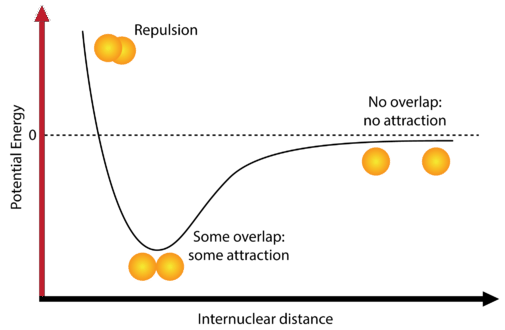# 9.1: Energy and Covalent Bond Formation

We learned that halide salts of elements in group 1 are typically ionic compounds. We would expect $$\ce{LiCl}$$ to exist as $$\ce{Li^+}$$ cations and $$\ce{Cl^-}$$ anions (and it does). However, if we move one column to the right, lithium's neighbor beryllium forms a different type of bond altogether. This bond consists of shared electrons between the $$\ce{Be}$$ and $$\ce{Cl}$$ atoms, instead of electrostatic attraction among ions.

## Energy and Covalent Bond Formation

Molecular compounds are those that take the form of an individual molecule. Molecular compounds are generally comprised of two or more nonmetal atoms. Familiar examples include water $$\left( \ce{H_2O} \right)$$, carbon dioxide $$\left( \ce{CO_2} \right)$$, and ammonia $$\left( \ce{NH_3} \right)$$. Recall that the molecular formula shows the number of each atom that occurs in a molecule of that compound. One molecule of water contains two hydrogen atoms and one oxygen atom. Hydrogen $$\left( \ce{H_2} \right)$$ is an example of an element that exists naturally as a diatomic molecule. A diatomic molecule is a molecule containing two atoms.

Most atoms attain a lower potential energy when they are bonded to other atoms than when they are separated. Consider two isolated hydrogen atoms that are separated by a distance large enough to prevent any interaction between them. At this distance, the potential energy of the system is said to be equal to zero (see figure below).Figure $$\PageIndex{1}$$: The graph shows how the potential energy of two hydrogen atoms changes as a function of their separation distance. (CC BY-NC; CK-12)

As the atoms approach one another, their electron clouds gradually begin to overlap. Now there are several interactions which begin to occur. One is that the single electrons that each hydrogen atom possesses begin to repel each other. This repulsive force would tend to make the potential energy of the system increase. However, the electron of each atom begins to be attracted to the nucleus of the other atom. This attractive force tends to make the potential energy of the system decrease.

As the atoms first begin to interact, the attractive force is stronger than the repulsive force and so the potential energy of the system decreases, as seen in the diagram. Remember that the lower potential energy increases the stability of the system. As the two hydrogen atoms move closer and closer together, the potential energy is at its lowest possible point. If the hydrogen atoms move any closer together, a third interaction begins to dominate, and that is the repulsive force between the two positively-charged nuclei. This repulsive force is very strong, as can be seen by the sharp rise in energy at the far left of the diagram.

The point at which the potential energy reached its minimum represents the ideal distance between hydrogen atoms for a stable chemical bond to occur. This type of chemical bond is called a covalent bond. A covalent bond is a bond in which two atoms share one or more pairs of electrons. The single electrons from each of the two hydrogen atoms are shared when the atoms come together to form a hydrogen molecule $$\left( \ce{H_2} \right)$$.

## Summary

• Molecular compounds are those that take the form of an individual molecule.
• A diatomic molecule is a molecule containing two atoms.
• Covalent bonds are formed when atoms share electrons between them.